## Topic 16.1: Simplex Method (Questions)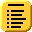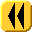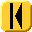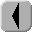Introduction Notes Theory HOWTO Examples Engineering Error Questions Matlab Maple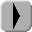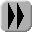# Question 1

Maximize f(x) = x1 + 2x2 subject to:

x1 + 2x2 ≤ 3
x1 + x2 ≤ 2
x1 ≤ 1
x1 ≥ 0
x2 ≥ 0

# Question 2

Maximize f(x) = x1 + 2x2 subject to:

x1 + 2x2 ≤ 5
x1 + x2 ≤ 4
2x1 + x2 ≤ 6
x1 ≥ 0
x2 ≥ 0

Solution: x = (4/3, 7/3)T.

# Question 3

Maximize f(x) = 2x1 + 3x2 + 2x3 subject to:

x1 + 2x2 + x3 ≤ 4
3x1 + x2 + x3 ≤ 5
x1 + x2 + 2x3 ≤ 4
x1 + x2 + x3 ≤ 3
x1 ≥ 0
x2 ≥ 0
x3 ≥ 0

# Question 4

Maximize f(x) = x1 + 2x2 + x3 subject to:

x1 + 2x2 + x3 ≤ 2
3x1 + x2 + x3 ≤ 4
x1 + x2 + 2x3 ≤ 4
x1 + x2 + x3 ≤ 2
x1 ≥ 0
x2 ≥ 0
x3 ≥ 0

Solution: x = (0, 0.4, 1.2)T.